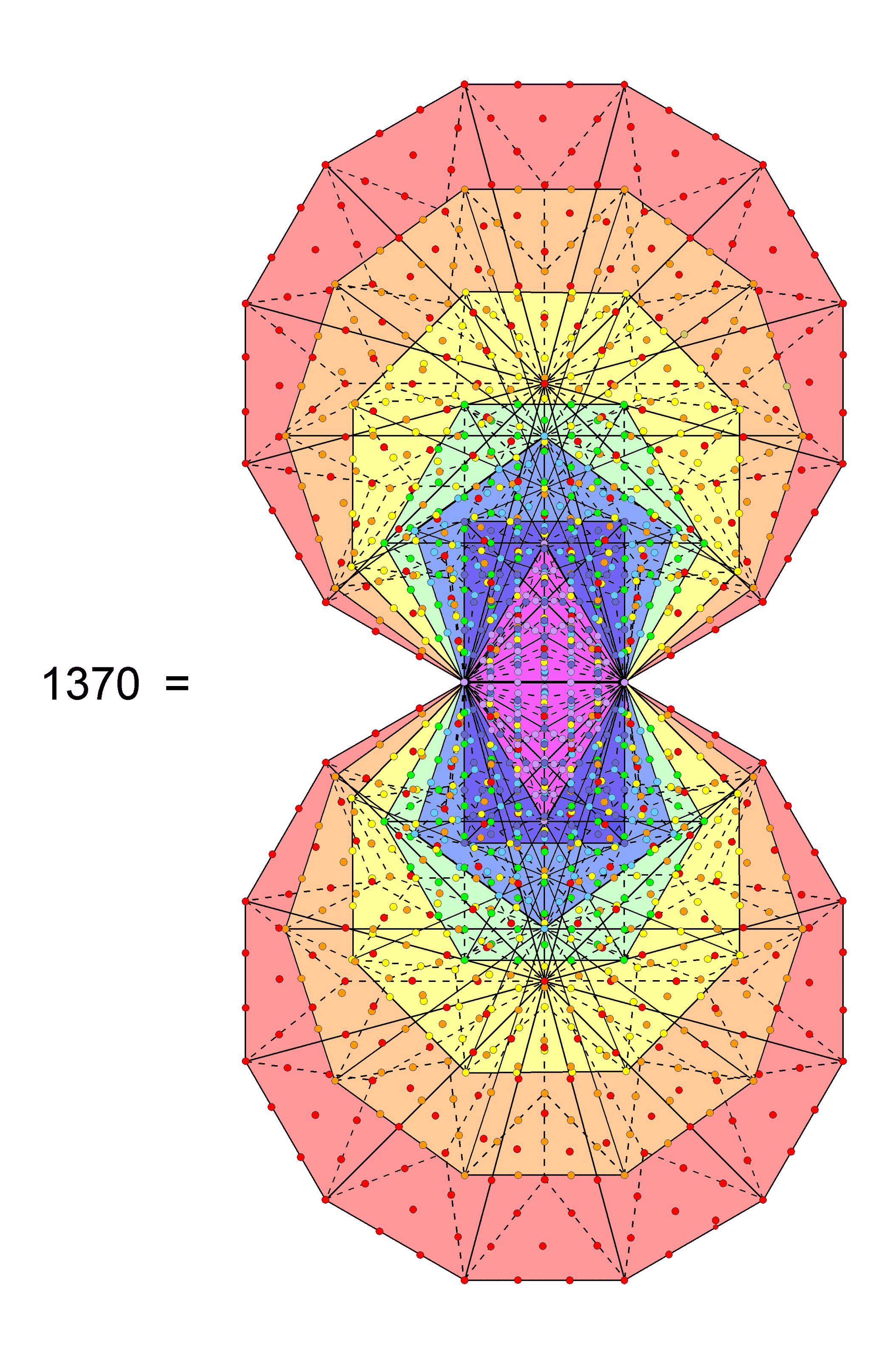<< Previous    1  2    4  5  ...36    Next >>

#3 The inner Tree of Life embodies the number 137 determining the fine-structure constantWhen the 94 sectors of the (7+7) enfolded, regular polygons of the inner Tree of Life are Type A triangles, they contain 1370 yods. This is the number of yods in 137 tetractyses. 137 is the 33rd prime number, where 33 = 1! + 2! + 3! + 4!. In atomic physics, the measure of the strength of the coupling of the electron to the electromagnetic field is the dimensionless fine-structure constant α = e2/ħc ≈ 1/137. Although it determines the magnitude of this constant only approximately, many physicists suspect that the number 137 will play a fundamental role in a "grand, unified theory" that integrates the forces of nature. In view of this, it should not be surprising that the number 137 is a defining parameter of sacred geometries (e.g., Platonic solids, disdyakis triacontahedron). Its manifestation in these geometries is most explicit in the inner form of the Tree of Life whose polygons are Type B. As the (7+7) enfolded polygons have 70 corners, 1300 more yods are needed to construct them from Type A triangles, where

 14 24 24 1300 = 15 + 25 + 35 + 45 = 34 34 34 44 44 44 44.

Such a beautiful property clearly indicates the archetypal nature of this sacred geometry. ADONAI, the Godname of Malkuth with number value 65, prescribes the 650 yods associated with each set of seven enfolded polygons because they contain the yods in 65 tetractyses (see also #17). As the 94 sectors of the (7+7) enfolded polygons have 80 corners, where 80 is the number of Yesod, they have 1290 (=129×10) more yods, showing how YAHWEH SABAOTH, the Godname of Netzach with number value 129, prescribes the inner Tree of Life.

Unlike those of the hexagon and decagon, the centres of the triangle, square, pentagon, octagon & dodecagon are not corners of other polygons. Each set of seven enfolded polygons, therefore, has five polygons with independent centres that, as yods, are intrinsic to them. There are (1370−5−5=1360) yods that surround the 10 independent centres of the (7+7) enfolded polygons. This is the sum of the gematria number values of Malkuth, its Godname ADONAI, its Archangel Sandalphon, its Order of Angels Ashim, and its Mundane Chakra Cholem Yesodeth:

496 + 65 + 280 + 351 + 168 = 1360.

As 1360 = 16×85, where 85 = 40 + 41 + 42 + 43 and 16 = 42,

1360 = 42(40 + 41 + 42 + 43) = 42 + 43 + 44 + 45

= 24 + 26 + 28 + 210.

Recognising that two yods in the root edge may be associated with one set of seven polygons and that the other two yods in the root edge may be associated with the second set, the number of yods associated with each set that surround its five independent centres = 1360/2 = 680 = 23 + 25 + 27 + 29. The mean number of such yods surrounding an independent centre = 680/5 = 136 = 8×17 = 1 + 2 + 3 + ...+ 16.

Three yods in each set of seven enfolded polygons are Sephirothic points shared with the outer Tree of Life: the topmost corner of the hexagon (Chokmah or Binah), one corner of the triangle (Chesed or Geburah) and the lowest corner of the hexagon (Netzach or Hod). The number of yods that are not such points = 1370 − 3 − 3 = 1364 = 4 + 1360 = 41 + 42 + 43 + 44 + 45 = 22 + 24 + 26 + 28 + 210. This is another beautiful property of the inner Tree of Life composed of Type B polygons (see also #34).

 << Previous    1  2    4  5  ...36    Next >>

Home